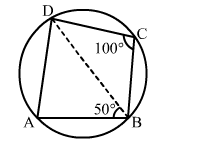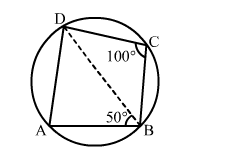# In the adjoining figure, ABCD is a cyclic quadrilateral in which ∠BCD = 100°

Question:

In the adjoining figure, ABCD is a cyclic quadrilateral in which BCD = 100° and ∠ABD = 50°. Find ∠ADB.Solution:Given: ABCD is a cyclic quadrilateral.
∴ DAB + DCB = 180°   ( Opposite angles of  a cyclic quadrilateral are supplementary)
⇒ DAB + 100° = 180°
⇒ DAB = (180° – 100°) = 80°
Now, in ΔABD, we have:
⇒ DAB + ABD + ADB = 180°
⇒ 80° + 50° + ADB = 180°
⇒ ADB = (180° – 130°) = 50°